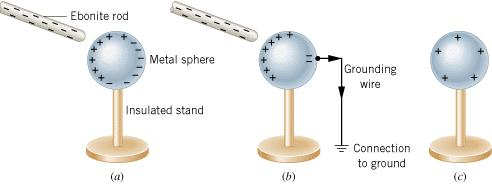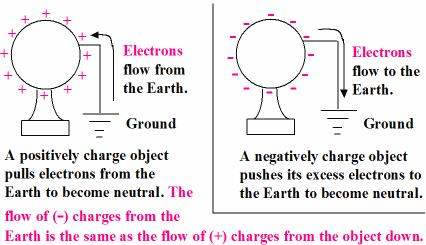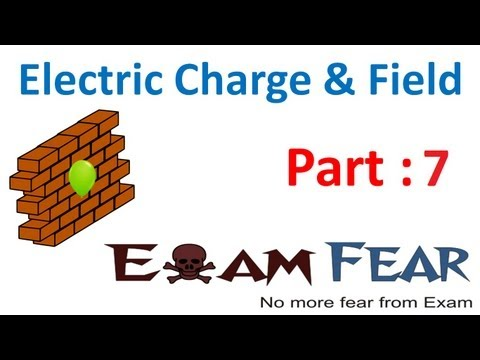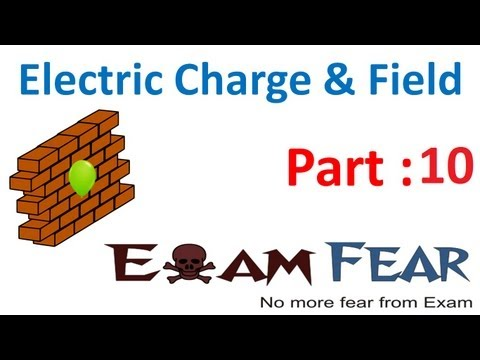Courses

Test: Electric Charge

20 Questions MCQ Test Physics For JEE | Test: Electric Charge

Description
Attempt Test: Electric Charge | 20 questions in 20 minutes | Mock test for JEE preparation | Free important questions MCQ to study Physics For JEE for JEE Exam | Download free PDF with solutions
QUESTION: 1

A material in which electrons are tightly bound and cannot move freely at room temperature is called _________.

Solution:

Insulators are those materials in which electron are tightly bound and cannot move freely at room temperature.

QUESTION: 2

According to the principle of conservation of charge, in closed system:

Solution:
• The law of conservation of charge states that electric charge can neither be created nor destroyed.
• In a closed system, the amount of charge remains the same. When something changes its charge, it doesn't create a charge but transfers it.
QUESTION: 3

A coulomb is same as:​

Solution:

► Current = Charge / Time

► Therefore Charge = Current. Time (unit of current = ampere, unit of time = second)

So, Charge = Ampere.Second

QUESTION: 4

When a glass rod is rubbed with silk, it becomes positively charged because:

Solution:
• The -ve charges of the glass rod get transferred to the silk cloth and hence itself becomes positively charged as there is the deficiency of -ve charge(electrons) on the glass rod due to their transfer.
• But the total charge of the system of rod and cloth remains zero.
QUESTION: 5

Electric charge between two bodies can be produced by:

Solution:

Electric charge between two bodies can be produced by rubbing with each other. The body having lower work function loses electron and becomes positive and the other body gains electrons become negative.
Example: When glass is rubbed with silk, glass becomes positive while silk becomes negative.

QUESTION: 6

Which of the following methods can be used to charge a metal sphere positively without touching it? Choose the best possible option:

Solution:

This can be possible by induction, if a negative charge rod is placed near the sphere (at a distance not in touch) than it attracts positive charge inside the sphere towards its side and the negative charges collected on other side and when the surface of sphere connected to earth than the negative charge goes to earth and the sphere remain positively charged.QUESTION: 7

If an object is positively charged, theoretically the mass of the object ______.

Solution:
• If an object is positively charged it loses some of its electrons.
• The mass of an electron is 9.11*10-31 kg.
• So, if a positively charged body loses ‘n’ number of electrons, it mass decrease by the amount n*9.11e-31kg.
QUESTION: 8

Choose the correct statement:

Solution:
• The theoretical reason for making the conclusion that the total charge of the universe is constant is the Law of Conservation of Charge.
• Because of certain symmetries in the structure of the universe, the total electric charge of an isolated system is always conserved. This means that the total charge of an isolated system is the same at all points in time.
QUESTION: 9

To make an uncharged object to have a negative charge we must:

Solution:

To make an uncharged object have a negative charge we must increase charge on particle and this can be done only by adding electrons to particle.

QUESTION: 10

When conductors are placed in an electric field, their electrons are moved:

Solution:

A substance is said to be a conductor if it conducts electricity. In the case of a conductor, the electrons are move in the opposite direction of the current, so it is able to conduct.

QUESTION: 11

When a negatively charged conductor is connected to earth,

Solution:

After earthing a positively charged conductor electrons flow from earth to conductor and if a negatively charged conductor is earthed then electrons flows from conductor to earth.QUESTION: 12

A negative ion is an atom that ________ electrons.

Solution:

Ions are formed when atoms gain or lose electrons. Gaining electrons changes an atom into a negative ion. Losing electrons changes an atom into a positive ion.

QUESTION: 13

When a hard rubber rod is given a negative charge by rubbing it with wool:

Solution:
• On rubbing an rubber rod with wool, the electrons from the fur get transferred to the rubber rod.
• The wool now has a deficiency of electrons and so is positively-charged.
• On the other hand, the rubber rod has an excess of electrons and hence is negatively-charged.
• In the above example, the wool undergoes positive electrification.
• It is to be noted that it is the electrons and not the protons that get transferred during positive (and negative) electrification.
• Here, the transfer of electrons and mass transfer respectively takes place from wool to the rubber rod.
QUESTION: 14

The value of charge on a body which carries 30 excess electrons is:

Solution:

Here, n = 30
► e = -1.6 x 10-19 C
So, q = n ∗ e = 30 * (1.6 x 10-19 C)
q = - 4.8 ∗ 10-18 C

QUESTION: 15

The total negative charge in 1 mol of helium (atomic number 2, atomic mass 4) is:

Solution:
• He atom has 2 electrons.
• So, 1 mole of He has 2*N(N is Avogadro's no.) electrons.
• Then total -ve charge in 1 mole He gas is =  2 * N * charge of 1 electron
= 2 * 6.022 * 1023 * 1.6 * 10-19 = 1.92 * 105
QUESTION: 16

When we rub a glass rod with silk, then:

Solution:
• When glass rod is rubbed with silk, glass happens to lose electrons easily and silk grabs them away from the glass atoms. So after rubbing, the glass becomes positively charged and the silk becomes negatively charged.
• Thus equal but opposite charge are produced on both. Hence, option D is correct.
QUESTION: 17

How many electrons are present in 1 coulomb of charge?​

Solution:

According to Quantisation of Charge we know that,
Q = ne
Therefore, 1 coulomb of Charge = n * 1.6 * 10-19
⇒ 1/ 1.6 × 10-19 = n
⇒ n = 6.25 × 1018

QUESTION: 18

What does q1 + q2 = 0 signify?​

Solution:

If we have two bodies with charge q1 and q2 on them and we are given q1 + q2 = 0. It means the net charge on the system is zero.

This is possible only if q1 and q2 are equal and opposite.

That is, q1 = +q and q2 = -q or vice versa.

Such that, q1 + q2 = +q + (-q) = 0

QUESTION: 19

A silk cloth rubbed with a glass rod acquire a charge (-1.6 x 10-19) C. Then the charge on the glass rod is​:

Solution:

During rubbing, glass rod acquires + ve charges and silk cloth acquires - ve charges.
∴ the charge on the glass rod is + 1.6×10- 19C

QUESTION: 20

To make uncharged object have a positive charge:

Solution:
• A body consists of atoms that have electrons, protons and neutrons. The electrons have a negative charge. The protons are positively charged and the neutrons are neutral.
• The atom is neutral because the positive and negative charges cancel out each other.
• On removing electrons, an excess of protons are present and thus the body is said to be positively charged.Use Code STAYHOME200 and get INR 200 additional OFF Use Coupon Code

Track your progress, build streaks, highlight & save important lessons and more!

Similar ContentRelated tests